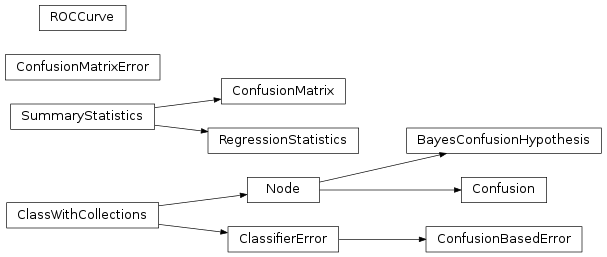# mvpa2.clfs.transerror¶

Utility class to compute the transfer error of classifiers.Functions

Classes

 `BayesConfusionHypothesis`([alpha, ...]) Bayesian hypothesis testing on confusion matrices. `ClassifierError`(clf[, labels, train]) Compute (or return) some error of a (trained) classifier on a dataset. `Confusion`([attr, labels, add_confusion_obj]) Compute a confusion matrix from predictions and targets (Node interface) `ConfusionBasedError`(clf[, labels, ...]) For a given classifier report an error based on internally computed error measure (given by some `ConfusionMatrix` stored in some conditional attribute of `Classifier`). `ConfusionMatrix`([labels, labels_map]) Class to contain information and display confusion matrix. `ConfusionMatrixError`([labels]) Compute confusion matrix as an “error function” `ROCCurve`(labels[, sets]) Generic class for ROC curve computation and plotting `RegressionStatistics`(**kwargs) Class to contain information and display on regression results. `SummaryStatistics`([targets, predictions, ...]) Basic class to collect targets/predictions and report summary statistics

Exceptions

 `BayesConfusionHypothesis`([alpha, ...]) Bayesian hypothesis testing on confusion matrices. `ClassifierError`(clf[, labels, train]) Compute (or return) some error of a (trained) classifier on a dataset. `Confusion`([attr, labels, add_confusion_obj]) Compute a confusion matrix from predictions and targets (Node interface) `ConfusionBasedError`(clf[, labels, ...]) For a given classifier report an error based on internally computed error measure (given by some `ConfusionMatrix` stored in some conditional attribute of `Classifier`). `ConfusionMatrix`([labels, labels_map]) Class to contain information and display confusion matrix. `ConfusionMatrixError`([labels]) Compute confusion matrix as an “error function” `ROCCurve`(labels[, sets]) Generic class for ROC curve computation and plotting `RegressionStatistics`(**kwargs) Class to contain information and display on regression results. `SummaryStatistics`([targets, predictions, ...]) Basic class to collect targets/predictions and report summary statistics